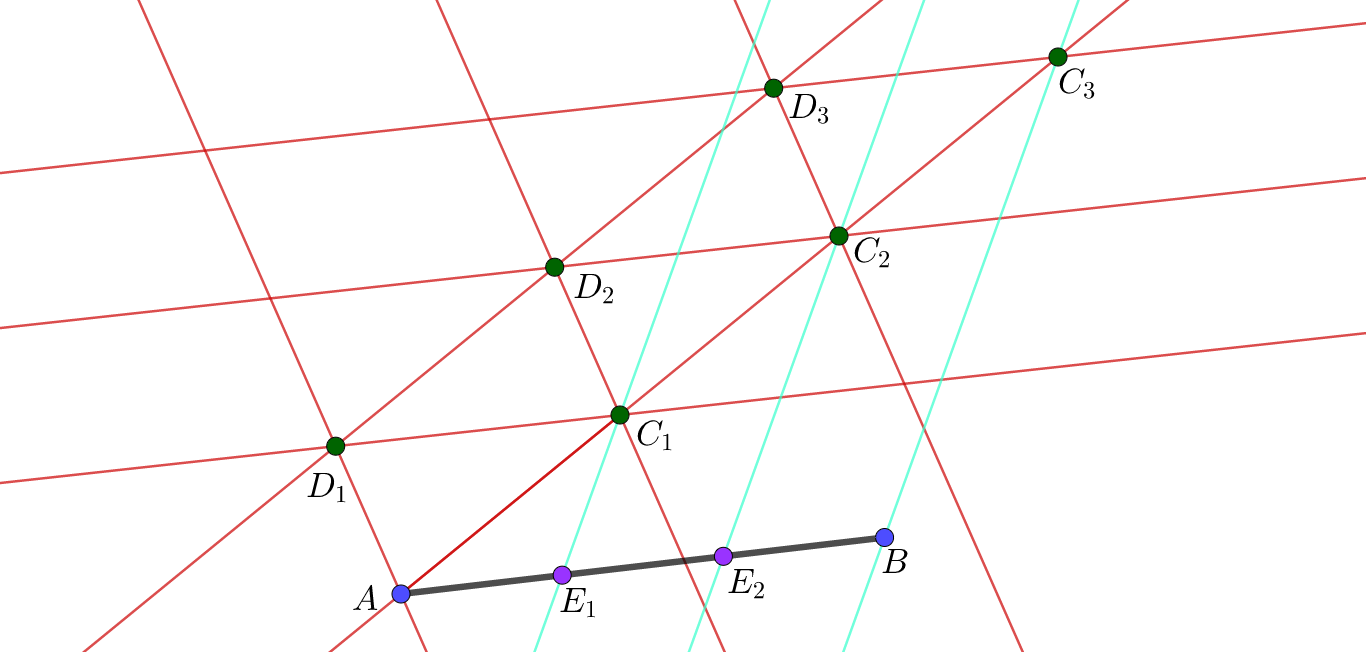# Dividing Line Segments in Affine Geometry

Here are the steps to divide a line segment into $n$ equal segments using only the tools in affine geometry. Here, we assume the axiom that there is a unique line through a given point that is parallel to a given line, i.e. the two lines do not meet.

1. Construct the line segment $\overline{AB}$, given points $A$ and $B$ in affine $n$-space.

2. Take one of the points on the line segment, say $A$, and draw a line through $A$ not parallel to the line $AB$.

3. Pick a point $C_1$ on the line constructed in Step 2.

4. Draw a line through $A$ not parallel to the line $AB$ or $AC_1$, and pick a point $D_1$ on this line.

5. Construct a parallel line to the line $AC_1$ through the point $D_1$.

6. Construct a parallel line to the line $AD_1$ through the point $C_1$, and define the meet (intersection) of this line with the line in Step 4 to be the point $D_2$.

7. Draw the line $D_1C_1$ and construct a parallel line to this line through the point $D_2$.

8. Define $C_2$ to be the meet of the line constructed in Step 6 with the line $AC_1$.

9. Repeat Steps 5-7, now with $C_1$, $C_2$ and $D_2$ instead of $A$, $C_1$ and $D_1$; we do this $n-1$ times, so that we get $n$ copies of the line segment $\overline{AC_1}$.

10. Suppose then that the last point we have is $C_n$; we then draw the line $BC_n$.

11. Through each of the points $C_i$, for $i$ ranging from $1$ to $n-1$, draw the lines through each of the points, parallel to the line drawn in Step 9.

12. The meets of each of the lines in Step 10 and the line segment $\overline{AB}$ will then be denoted by $E_i$, for $i$ ranging from $1$ to $n-1$.

As a result of this construction, we then have that the points $E_i$, for $i$ ranging from $1$ to $n-1$, divide the line segment $\overline{AB}$ into $n$ equal line segments.I did a construction (see picture above) where I just divided a simple line segment into $3$ equal segments.

EDIT: Picture too small to look at by eye; not sure how to make it bigger. Just click to enlarge, I guess.

EDIT 2: There are a lot more things to say about this, but I am going to write a book with this content in it. Don't want to spoil too much...Note by A Former Brilliant Member
2 years, 11 months ago

This discussion board is a place to discuss our Daily Challenges and the math and science related to those challenges. Explanations are more than just a solution — they should explain the steps and thinking strategies that you used to obtain the solution. Comments should further the discussion of math and science.

When posting on Brilliant:

• Use the emojis to react to an explanation, whether you're congratulating a job well done , or just really confused .
• Ask specific questions about the challenge or the steps in somebody's explanation. Well-posed questions can add a lot to the discussion, but posting "I don't understand!" doesn't help anyone.
• Try to contribute something new to the discussion, whether it is an extension, generalization or other idea related to the challenge.

MarkdownAppears as
*italics* or _italics_ italics
**bold** or __bold__ bold
- bulleted- list
• bulleted
• list
1. numbered2. list
1. numbered
2. list
Note: you must add a full line of space before and after lists for them to show up correctly
paragraph 1paragraph 2

paragraph 1

paragraph 2

[example link](https://brilliant.org)example link
> This is a quote
This is a quote
    # I indented these lines
# 4 spaces, and now they show
# up as a code block.

print "hello world"
# I indented these lines
# 4 spaces, and now they show
# up as a code block.

print "hello world"
MathAppears as
Remember to wrap math in $$ ... $$ or $ ... $ to ensure proper formatting.
2 \times 3 $2 \times 3$
2^{34} $2^{34}$
a_{i-1} $a_{i-1}$
\frac{2}{3} $\frac{2}{3}$
\sqrt{2} $\sqrt{2}$
\sum_{i=1}^3 $\sum_{i=1}^3$
\sin \theta $\sin \theta$
\boxed{123} $\boxed{123}$